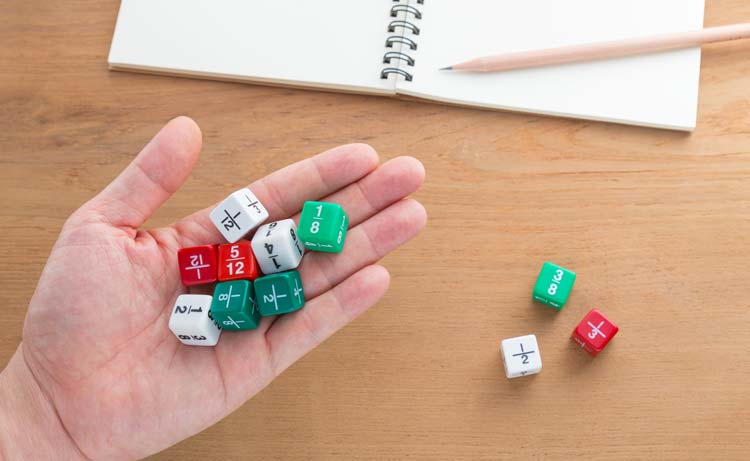# How To Add, Subtract, Multiply And Divide Fractions

| Last update: 25 February 2020

While some people might be breathing deeply into a paper bag at the thought of calculating with fractions, if you understand each step and why it's necessary, it can become a piece of cake. Or 1/6 of a cake, if you like.

A rule to remember:

Whether you're adding, subtracting, multiplying or dividing fractions, you should always aim for the "tidiest" answer possible. Tell your fraction if "its bum looks big in this" and simplify it down to the smallest option (e.g. 3/6 becomes 1/2, which retains the same proportions but is less clumsy).## How to add and subtract fractions

1. Check: do your denominators match?
2. If not, multiply them together (and balance the numerators accordingly)
3. Add or subtract using the numerators, keeping the denominator the same.

For an adding or subtracting task, you need to make "common denominators". The hardest thing about common denominators is trying to say it. Go on, recite it quickly ten times and come back to read the rest of the article when you've finished crying.

OK? Good.

### What are denominators and numerators?

A denominator is the number hanging out below the fraction line, so "common denominator" just means that you need all those numbers in the sum to be the same as each other. The number sitting on top of the fraction line is called the numerator.

When it comes to adding and subtracting fractions, it's very simple once you've checked for (or created, if necessary) a common denominator.

### Step 1) Check: do your denominators match?

If you're very lucky, your denominators will already be the same, so you just add together the numerators from the top halves and keep your existing denominator under the line.

Example: 1/4 + 1/4 = 2/4

... And it's the same deal for a subtraction sum, except you subtract the numerators instead:

Example: 2/3 - 1/3 = 1/3

### Step 2) If denominators do not match

If the denominators are not already the same number (like if you want to add 1/4 + 2/3), you'll need to multiply them together. The figure you get from the multiplication becomes the common denominator, and therefore forms the bottom part of each fraction in the sum.

Example: If you need to do 1/4 + 2/3, make a common denominator:

4 x 3 = 12. Now 12 goes on the bottom of each fraction.

Now you're working with the same number under each fraction. And of course, you need to adjust the numerators accordingly, or your fractions won't be equivalent. Think about it...If you paid for 1/4 of a cheesecake, you would be furious - and hungry - if you were just handed 1/12 of the cheesecake. Those aren't equivalent, so you need to adjust the numerator so it's proportionately the same size.

You do this by multiplying the numerator by the same figure you used to multiply the denominator. Look:

1/4 becomes 3/12 (both parts, top and bottom, have been multiplied by 3)

And 2/3 becomes 8/12 (both parts, top and bottom, have been multiplied by 4)

So, you've made sure that there's a common denominator, and that nobody's ripped you off with a smaller piece of cake (i.e. you adjusted your numerator accordingly). Now you're now free to do your simple sum, adding or subtracting, using the numerators. Leave your common denominator as it is; that doesn't change now. That's it!

Example: SUM: 1/4 + 2/3

Multiply your denominators together (4 x 3 = 12), and adjust the numerators proportionately: 3/12 + 8/12

Do the sum on top of the fraction = 11/12

Does my bum look big in this? Nope. This is the smallest this fraction can be.

Let's try another example...

Example: SUM: 3/4 - 1/8

Multiply your denominators together (4 x 8 = 32), and adjust the numerators proportionately: 24/32 - 4/32

Do the sum on top of the fraction = 20/32

Does my bum look big in this? Sorry, yeah it does a bit. 20/32 can be simplified down to 5/8. Much better!

## How to multiply fractions

When you want to multiply fractions together, it's beautifully simple.

1. Multiply the numerators together: anything sitting on top of the fraction gets multiplied together. This becomes the numerator of your answer.
2. Multiply the denominators together: anything lurking below the line in the fraction gets multiplied together. This becomes the denominator in your answer.
3. Does my bum look big in this? Shrink the fraction to the smallest denominator possible.

Example: 4/5 x 1/4 = 4/20 = simplified is 1/5

## How to divide fractions

[TIP] When dividing fractions, you don't ever have to do any division. Weird, right? Instead, we are going to multiply. It makes more sense if you consider that division is the opposite of multiplication, so we flip one of the fractions upside down to compensate for this.

### Step 1

The first thing you want to do is make it a multiplication sum. Get rid of that divide symbol and replace with an X.

But now it's a totally different sum, right? It's going to make a bigger number instead of a smaller one? Aha! Well...

### Step 2

Flip the second fraction upside down, putting the old denominator on TOP of the line and the numerator underneath.

### Example:

Let's say you have the following fraction sum:

1/2 ÷ 3/4

Keep the first fraction the same:

1/2

Change the ÷ to x:
1/2 x 3/4

And flip the second fraction upside down.

1/2 x 4/3

So your calculation (and you know how to multiply from the previous section) is:

1/2 ÷ 3/4
which becomes
1/2 x 4/3
= 2/3

Does my bum look big in this? No, you look wonderful, 2/3! You're perfectly simple as you are.